## Hard Question

A stone is dropped into a well. t seconds later, a splash is heard. The acceleration due to gravity is g metres per second per second, and the speed of sound is c metres per second. Calculate the depth of the well and the downwards velocity with which the stone hits the water.

### Input Format

Line 1: The value of g (0 < g ≤ 1000)

Line 2: The value of c (0 < c ≤ 1000)

Line 3: The value of t (0 < t ≤ 1000)

### Output Format

Line 1: The depth of the well in metres.

Line 2: The speed of the stone as it hits the water, in metres per second.

### Sample Input

9.80
334
3.41

### Sample Output

51.90
31.90

Your answers must have a relative or absolute error of less than 0.01.

### Notes

The following equations may be useful:

•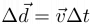$\Delta \vec{d} = \vec{v} \Delta t$
•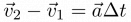$\vec{v}_2 - \vec{v}_1 = \vec{a} \Delta t$
•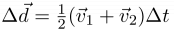$\Delta \vec{d} = \frac{1}{2} (\vec{v}_1 + \vec{v}_2) \Delta t$
•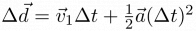$\Delta \vec{d} = \vec{v}_1 \Delta t + \frac{1}{2} \vec{a}(\Delta t)^2$
•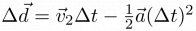$\Delta \vec{d} = \vec{v}_2 \Delta t - \frac{1}{2} \vec{a}(\Delta t)^2$
•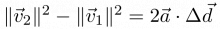$\|\vec{v}_2\|^2 - \|\vec{v}_1\|^2 = 2\vec{a}\cdot \Delta \vec{d}$

Here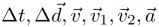$\Delta t, \Delta \vec{d}, \vec{v}, \vec{v}_1, \vec{v}_2, \vec{a}$ represent time, displacement, velocity, initial velocity, final velocity, and acceleration, respectively.

Point Value: 3
Time Limit: 2.00s
Memory Limit: 16M
Author: bbi5291

Problem Types: [Show]

Languages Allowed:
C++03, PAS, C, HASK, ASM, RUBY, PYTH2, JAVA, PHP, SCM, CAML, PERL, C#, C++11, PYTH3

• (0/0)
Looks like the judge was wrong...

• (0/0)
yeah, its because of the rounding error, jargon manually most of the submissions, but its still not fixed yet

• (0/0)
Actually, it has been fixed, so manual rejudging shouldn't be necessary now.

• (1/0)
my physics seems right, but why am i getting WA? can you please tell me if its a formating error/round error of algorithm error, help me please, thank you!

• (1/0)
rounding or algorithm error

• (1/0)

2. Our sincerest apologies. This problem was effectively impossible for a while, due to an error on our end. I have resolved this error, and I manually rejudged several affected submissions (at least one from each user who posted since the mistake); the number of accepted users has increased from 49 to 63.

Please resubmit if I missed you or you believe you may have been affected.

• (0/0)
What does per second per second mean?
 The acceleration due to gravity is g metres per second per second

• (0/0)
This will make sense once you've had exposure to elementary physics.

• (0/1)
per second per second?
it repeats twice!

• (0/0)
the short of it:

how fast you go is change in displacement, measured in [metres] per second. (velocity.)
how fast you're increasing how fast you go is change in velocity, measured in [metres per second] per second. (acceleration.)

• (1/1)
I'm not sure why my program doesn't work

• (1/1)
You are experiencing significant loss of precision.

Edit: I was wrong. Your code was right, and the judge was wrong. I apologise.

• (0/0)
OK, so I tried all sorts of rounding methods, but I keep getting the wrong answer. In the sample input, my program rounds the speed of the stone to 31.93. How was the sample input rounded, because the full answer is around 31.929799, yet the sample output says only 31.90?

• (1/1)
51.9029278687
31.8950997212

• (0/1)
How are you supposed to do this questions? How come it's so hard when it's only worth 3 points? If anybody knows, can they give me the formuala in words that I can actually UNDERSTAND? THANK YOU!!!

• (0/0)
It's not hard; it just requires a basic understanding of high school algebra and physics. The point value stands. (I even gave you the equations of kinematics you need to solve this!)

• (0/0)
Well then could you tell me the meaning of the equations? It's so hard to understand when your only in grade 7.

• (0/0)
These equations describe uniformly accelerated linear motion, where Δt is elapsed time, Δd is displacement, v₁ is initial velocity, v₂ is final velocity, and a is acceleration.

• (0/0)
I recommend simply not doing this question until you've taken Grade 11 Physics.

• (0/0)

• (0/0)
LOL.
No need for physics here, I just BF'ed.
Digital technology's so great (compared to analog) as you can just set an arbitrary precision depending on how precise the situation needs (with the equivalent time costs, of course).

• (0/0)
I can't find anything wrong in my equation.

• (0/0)
I haven't read the problem, so I can't comment on who's doing what right, but Sour Spinach has a different algorithm to get 5/5. Notice the time his solution took.

Edit: But, according to the analysis, it's not necessary. So I don't actually know what's going on.

• (2/0)
Aah, you don't know how close you are! You forgot to divide by 2 when using the fourth equation, most likely.

• (0/0)
THANK YOU SO MUCH

• (1/0)
How do people in Grade 6-7 do this program (how is it only 2 points!!!)? We don't know anything about this physics stuff (or something like that).

• (1/0)
It's for didactic purposes only. Although, since, when I think of it, the same can be said for all problems on the judge, I guess it should be 3 points, since it's harder than A plus B, and around the difficulty of an easy CCC problem.

• (0/0)
Thanks for the equations!